# RD Sharma solutions for Mathematics for Class 9 chapter 15 - Circles [Latest edition]

#### Chapters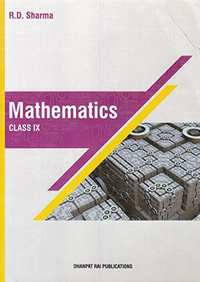## Chapter 15: Circles

Exercise 15.1Exercise 15.2Exercise 15.3Exercise 15.4Exercise 15.5Exercise 15.6Exercise 15.7
Exercise 15.1 [Pages 5 - 6]

### RD Sharma solutions for Mathematics for Class 9 Chapter 15 Circles Exercise 15.1 [Pages 5 - 6]

Exercise 15.1 | Q 1.01 | Page 5

Fill in the blank:

All points lying inside/outside a circle are called .................. points/ .....................points.

Exercise 15.1 | Q 1.02 | Page 5

Fill in the blank

Circles having the same centre and different radii are called ...........................circles.

Exercise 15.1 | Q 1.03 | Page 5

Fill in the blank:

A point whose distance from the centre of a circle is greater than its radius lies in ..................... of the circle.

Exercise 15.1 | Q 1.04 | Page 5

Fill in the blank

A continuous piece of a circle is ............... of the circle

Exercise 15.1 | Q 1.05 | Page 5

Fill in the blank:

The longest chord of a circle is a ................of the circle.

Exercise 15.1 | Q 1.06 | Page 5

Fill in the blank:

An arc is a ................ when its ends are the ends of a diameter.

Exercise 15.1 | Q 1.07 | Page 5

Fill in the blank:

Segment of a circle is the region between an arc and .................. of the circle.

Exercise 15.1 | Q 1.08 | Page 5

Fill in the blank:

A circle divides the plane, on which it lies, in ............ parts.

Exercise 15.1 | Q 2.01 | Page 6

true or false

A circle is a plane figure.

Exercise 15.1 | Q 2.02 | Page 6

true or false

Line segment joining the centre to any point on the circle is a radius of the circle,

Exercise 15.1 | Q 2.03 | Page 6

true or false

If a circle is divided into three equal arcs each is a major arc.

Exercise 15.1 | Q 2.04 | Page 6

true or false

A circle has only finite number of equal chords.

Exercise 15.1 | Q 2.05 | Page 6

true or false

A chord of a circle, which is twice as long is its radius is a diameter of the circle.

Exercise 15.1 | Q 2.06 | Page 6

true or false

Sector is the region between the chord and its corresponding arc.

Exercise 15.1 | Q 2.07 | Page 6

true or false

The degree measure of an arc is the complement of the central angle containing the arc.

Exercise 15.1 | Q 2.08 | Page 6

ture or false v

The degree measure of a semi-circle is 180°.

Exercise 15.2 [Pages 28 - 29]

### RD Sharma solutions for Mathematics for Class 9 Chapter 15 Circles Exercise 15.2 [Pages 28 - 29]

Exercise 15.2 | Q 1 | Page 28

The radius of a circle is 8 cm and the length of one of its chords is 12 cm. Find the distance of the chord from the centre.

Exercise 15.2 | Q 2 | Page 28

Find the length of a chord which is at a distance of 5 cm from the centre of a circle ofradius 10 cm.

Exercise 15.2 | Q 3 | Page 28

Find the length of a chord which is at a distance of 4 cm from the centre of the circle of radius 6 cm.

Exercise 15.2 | Q 5 | Page 28

Give a method to find the centre of a given circle.

Exercise 15.2 | Q 6 | Page 29

A line segment AB is of length 5 cm. Draw a circle of radius 4 cm passing through A and B. Can you draw a circle of radius 2 cm passing through A and B? Give reason in support of your answer.

Exercise 15.2 | Q 7 | Page 28

Prove that a diameter of a circle which bisects a chord of the circle also bisects the angle subtended by the chord at the centre of the circle.

Exercise 15.2 | Q 7 | Page 29

An equilateral triangle of side 9cm is inscribed in a circle. Find the radius of the circle.

Exercise 15.2 | Q 8

Given an arc of a circle, show how to complete the circle.

Exercise 15.2 | Q 9 | Page 29

Given an arc of a circle, complete the circle.

Exercise 15.2 | Q 10 | Page 29

Suppose You Are Given a Circle. Give a Construction to Find Its Centre.

Exercise 15.2 | Q 11 | Page 29

The lengths of two parallel chords of a circle are 6 cm and 8 cm. if the smaller chord is at a distance of 4 cm from the centre, what is the distance of the other chord from the centre?

Exercise 15.2 | Q 12 | Page 29

Two chords AB, CD of lengths 5 cm, 11 cm respectively of a circle are parallel. If the distance between AB and CD is 3 cm, find the radius of the circle.

Exercise 15.2 | Q 13

Draw different pairs of circles. How many points does each pair have in common? What is the maximum number of common points?

Exercise 15.2 | Q 13 | Page 29

Prove that the line joining the mid-point of a chord to the centre of the circle passes through the mid-point of the corresponding minor arc.

Exercise 15.2 | Q 14 | Page 29

Prove that two different circles cannot intersect each other at more than two points.

Exercise 15.2 | Q 15 | Page 29

Two chords AB and CD of lengths 5 cm and 11 cm respectively of a circle are parallel to each other and are opposite side of its center. If the distance between AB and CD is 6 cm. Find the radius of the circle.

Exercise 15.3 [Page 47]

### RD Sharma solutions for Mathematics for Class 9 Chapter 15 Circles Exercise 15.3 [Page 47]

Exercise 15.3 | Q 1 | Page 47

Three girls Ishita, Isha and Nisha are playing a game by standing on a circle of radius 20 m drawn in a park. Ishita throws a ball o Isha, Isha to Nisha and Nisha to Ishita. If the distance between Ishita and Isha and between Isha and Nisha is 24 m each, what is the distance between Ishita and Nisha.

Exercise 15.3 | Q 2 | Page 47

A circular park of radius 40 m is situated in a colony. Three boys Ankur, Amit and Anand are sitting at equal distance on its boundary each having a toy telephone in his hands to talk
to each other.

Exercise 15.4 [Pages 72 - 74]

### RD Sharma solutions for Mathematics for Class 9 Chapter 15 Circles Exercise 15.4 [Pages 72 - 74]

Exercise 15.4 | Q 1 | Page 72

In the below fig. O is the centre of the circle. If ∠APB = 50°, find ∠AOB and ∠OAB.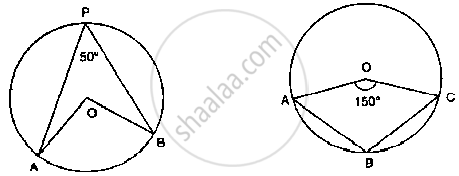Exercise 15.4 | Q 2 | Page 72

In the below fig. O is the centre of the circle. Find ∠BAC.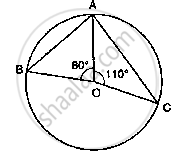Exercise 15.4 | Q 3.01 | Page 72

If O is the centre of the circle, find the value of x in the following figure: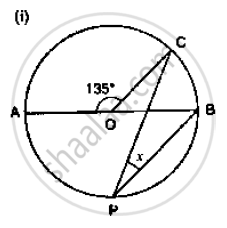Exercise 15.4 | Q 3.02 | Page 72

If O is the centre of the circle, find the value of x in the following figure: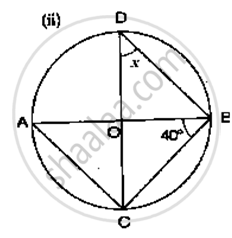Exercise 15.4 | Q 3.03 | Page 72

If O is the centre of the circle, find the value of x in the following figure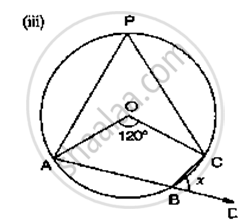Exercise 15.4 | Q 3.04 | Page 72

If O is the centre of the circle, find the value of x in the following figure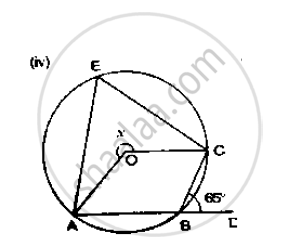Exercise 15.4 | Q 3.05 | Page 72

If O is the centre of the circle, find the value of x in the following figure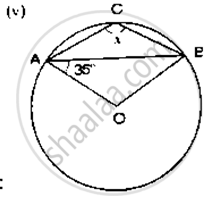Exercise 15.4 | Q 3.06 | Page 72

If O is the centre of the circle, find the value of x in the following figure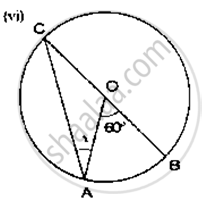Exercise 15.4 | Q 3.07 | Page 72

If O is the centre of the circle, find the value of x in the following figure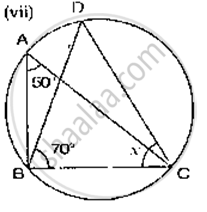Exercise 15.4 | Q 3.08 | Page 72

If O is the centre of the circle, find the value of x in the following figure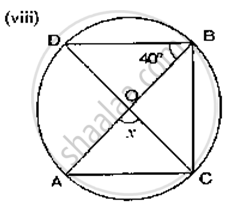Exercise 15.4 | Q 3.09 | Page 72

If O is the centre of the circle, find the value of x in the following figure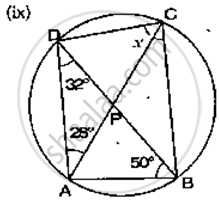Exercise 15.4 | Q 3.1 | Page 72

If O is the centre of the circle, find the value of x in the following figure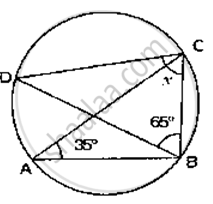Exercise 15.4 | Q 3.11 | Page 72

If O is the centre of the circle, find the value of x in the following figures.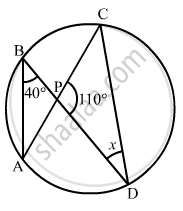Exercise 15.4 | Q 3.12 | Page 72

If O is the centre of the circle, find the value of x in the following figures.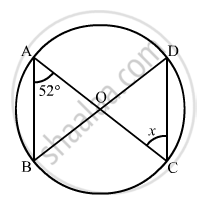Exercise 15.4 | Q 4 | Page 73

O is the circumcentre of the triangle ABC and OD is perpendicular on BC. Prove that ∠BOD = ∠A

Exercise 15.4 | Q 5 | Page 73

In the given figure, O is the centre of the circle, BO is the bisector of ∠ABC. Show that AB = BC.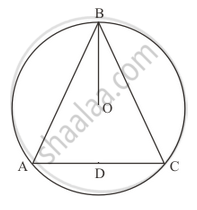Exercise 15.4 | Q 6 | Page 73

In the given figure, O and O' are centres of two circles intersecting at B and CACD is a straight line, find x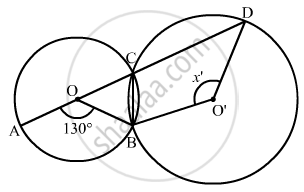Exercise 15.4 | Q 7 | Page 74

In the given figure, if ∠ACB = 40°, ∠DPB = 120°, find ∠CBD.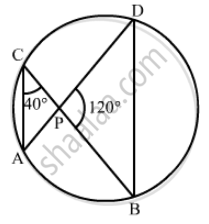Exercise 15.4 | Q 8

A chord of a circle is equal to the radius of the circle. Find the angle subtended by the chord at a point on the minor arc and also at a point on the major arc.

Exercise 15.4 | Q 9 | Page 74

In the given figure, it is given that O is the centre of the circle and ∠AOC = 150°. Find ∠ABC.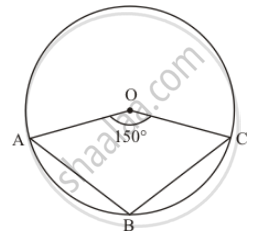Exercise 15.4 | Q 10 | Page 74

In the given figure, is the centre of the circle, prove that ∠x = ∠y + ∠z.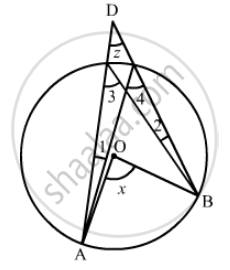Exercise 15.4 | Q 11 | Page 74

In the given figure, O is the centre of a circle and PQ is a diameter. If ∠ROS = 40°, find ∠RTS.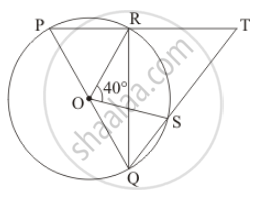Exercise 15.5 [Pages 100 - 104]

### RD Sharma solutions for Mathematics for Class 9 Chapter 15 Circles Exercise 15.5 [Pages 100 - 104]

Exercise 15.5 | Q 1 | Page 100

In the given figure, ΔABC is an equilateral triangle. Find mBEC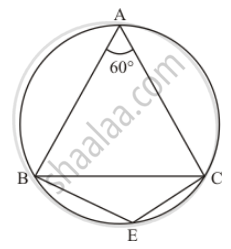Exercise 15.5 | Q 2 | Page 100

In the given figure, ΔPQR is an isosceles triangle with PQ = PR and m ∠PQR = 35°. Find m ∠QSR and QTR.Exercise 15.5 | Q 3 | Page 101

In the given figure, O is the centre of the circle. If ∠BOD = 160°, find the values of x and y.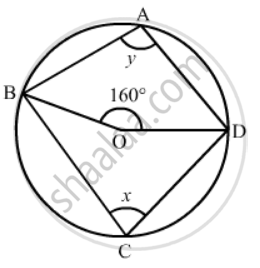Exercise 15.5 | Q 4 | Page 101

In the given figure, ABCD is a cyclic quadrilateral. If ∠BCD = 100° and ∠ABD = 70°, find ∠ADB.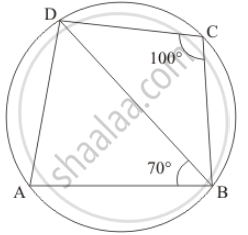Exercise 15.5 | Q 5 | Page 101

If ABCD is a cyclic quadrilateral in which AD || BC (In the given figure). Prove that ∠B = ∠C.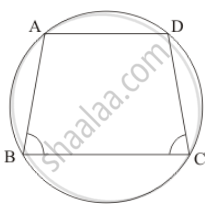Exercise 15.5 | Q 6 | Page 101

In the given figure, is the centre of the circle. Find ∠CBD.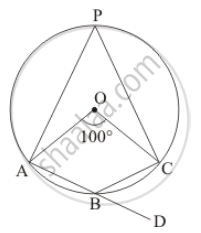Exercise 15.5 | Q 7 | Page 101

In the given figure, AB and CD are diameters of a circle with centre O. If ∠OBD = 50°, find ∠AOC.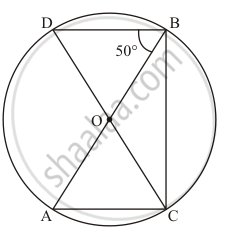Exercise 15.5 | Q 8 | Page 101

On a semi-circle with AB as diameter, a point C is taken, so that m (∠CAB) = 30°. Find m(∠ACB) and m (∠ABC).

Exercise 15.5 | Q 9 | Page 101

In a cyclic quadrilateral ABCD if AB || CD and ∠B = 70°, find the remaining angles.

Exercise 15.5 | Q 10 | Page 101

In a cyclic quadrilateral ABCD, if A = 3 (m ∠C). Find m ∠A.

Exercise 15.5 | Q 11 | Page 102

In the given figure, O is the centre of the circle and ∠DAB = 50° . Calculate the values of xand y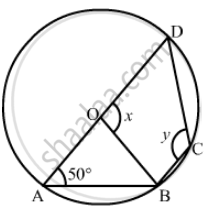Exercise 15.5 | Q 12 | Page 102

In the given figure, if ∠BAC = 60° and ∠BCA = 20°, find ∠ADC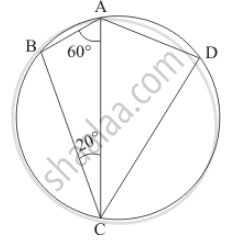Exercise 15.5 | Q 13 | Page 102

In the given figure, if ABC is an equilateral triangle. Find ∠BDC and ∠BEC.Exercise 15.5 | Q 14 | Page 102

In the given figure, O is the centre of the circle. If ∠CEA = 30°, Find the values of xy and z.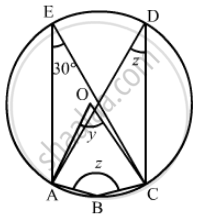Exercise 15.5 | Q 15 | Page 102

In the given figure, ∠BAD = 78°, ∠DCF = x° and ∠DEF = y°. Find the values of x and y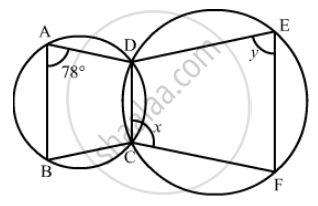Exercise 15.5 | Q 16 | Page 102

In a cyclic quadrilateral ABCD, if ∠A − ∠C = 60°, prove that the smaller of two is 60°

Exercise 15.5 | Q 17 | Page 103

In the given figure, ABCD is a cyclic quadrilateral. Find the value of x.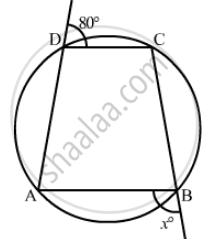Exercise 15.5 | Q 18.1 | Page 103

ABCD is a cyclic quadrilateral in  BC || AD, ∠ADC = 110° and ∠BAC = 50°. Find ∠DAC.

Exercise 15.5 | Q 18.2 | Page 103

ABCD is a cyclic quadrilateral in  ∠DBC = 80° and ∠BAC = 40°. Find ∠BCD.

Exercise 15.5 | Q 18.3 | Page 103

ABCD is a cyclic quadrilateral in   ∠BCD = 100° and ∠ABD = 70° find ∠ADB.

Exercise 15.5 | Q 19 | Page 103

Prove that the circles described on the four sides of a rhombus as diameters, pass through the point of intersection of its diagonals.

Exercise 15.5 | Q 20 | Page 103

If the two sides of a pair of opposite sides of a cyclic quadrilateral are equal, prove that its diagonals are equal.

Exercise 15.5 | Q 21 | Page 103

Circles are described on the sides of a triangle as diameters. Prove that the circles on any two sides intersect each other on the third side (or third side produced).

Exercise 15.5 | Q 22 | Page 103

ABCD is a cyclic trapezium with AD || BC. If ∠B = 70°, determine other three angles of the trapezium.

Exercise 15.5 | Q 23 | Page 103

In the given figure, ABCD is a cyclic quadrilateral in which AC and BD are its diagonals. If ∠DBC = 55° and ∠BAC = 45°, find ∠BCD.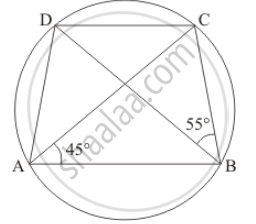Exercise 15.5 | Q 24 | Page 103

Prove that the perpendicular bisectors of the sides of a cyclic quadrilateral are concurrent.

Exercise 15.5 | Q 25 | Page 103

Prove that the centre of the circle circumscribing the cyclic rectangle ABCD is the point of intersection of its diagonals.

Exercise 15.5 | Q 26.1 | Page 103

ABCD is a cyclic quadrilateral in which BA and CD when produced meet in E and EA = ED. Prove that AD || BC .

Exercise 15.5 | Q 26.2 | Page 103

ABCD is a cyclic quadrilateral in which BA and CD when produced meet in E and EA = ED. Prove that  EB = EC

Exercise 15.5 | Q 27 | Page 104

Prove that the angle in a segment shorter than a semicircle is greater than a right angle.

Exercise 15.5 | Q 28 | Page 104

Prove that the angle in a segment greater than a semi-circle is less than a right angle.

Exercise 15.5 | Q 29 | Page 104

Prove that the line segment joining the mid-point of the hypotenuse of a right triangle to its opposite vertex is half the hypotenuse.

Exercise 15.6 [Pages 107 - 109]

### RD Sharma solutions for Mathematics for Class 9 Chapter 15 Circles Exercise 15.6 [Pages 107 - 109]

Exercise 15.6 | Q 1 | Page 107

In the given figure, two circles intersect at A and B. The centre of the smaller circle is Oand it lies on the circumference of the larger circle. If ∠APB = 70°, find ∠ACB.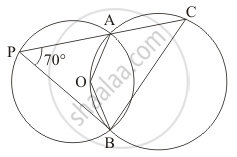Exercise 15.6 | Q 2 | Page 108

In the given figure, two congruent circles with centres O and O' intersect at A and B. If ∠AOB = 50°, then find ∠APB.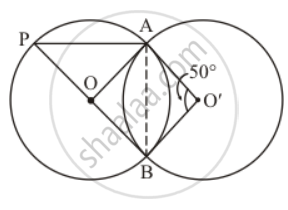Exercise 15.6 | Q 3 | Page 108

In the given figure, ABCD is a cyclic quadrilateral in which ∠BAD = 75°, ∠ABD = 58° and ∠ADC = 77°, AC and BD intersect at P. Then, find ∠DPC.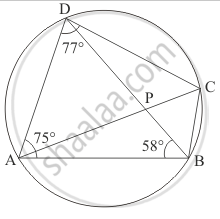Exercise 15.6 | Q 4 | Page 108

In the given figure, if ∠AOB = 80° and ∠ABC = 30°, then find ∠CAO.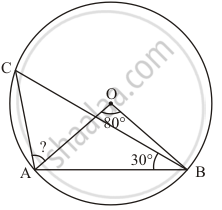Exercise 15.6 | Q 5 | Page 108

In the given figure, A is the centre of the circle. ABCD is a parallelogram and CDE is a straight line. Find ∠BCD : ∠ABE.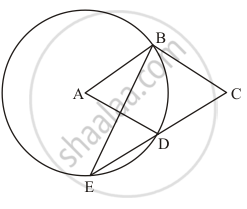Exercise 15.6 | Q 6 | Page 108

In the given figure, AB is a diameter of the circle such that ∠A = 35° and ∠Q = 25°, find ∠PBR.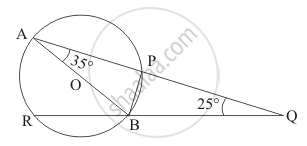Exercise 15.6 | Q 7 | Page 108

In the given figure, P and Q are centres of two circles intersecting at B and CACD is a straight line. Then, ∠BQD =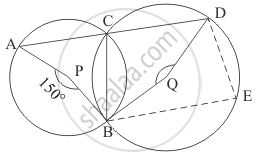Exercise 15.6 | Q 8 | Page 109

In the given figure, if O is the circumcentre of ∠ABC, then find the value of ∠OBC + ∠BAC.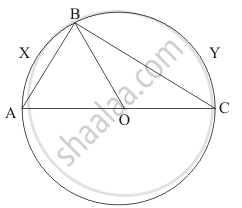Exercise 15.6 | Q 9 | Page 109

If the given figure, AOC is a diameter of the circle and arc AXB = $\frac{1}{2}$ arc BYC. Find ∠BOC.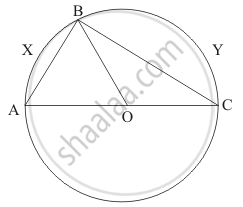Exercise 15.6 | Q 10 | Page 109

In the given figure, ABCD is a quadrilateral inscribed in a circle with centre OCD is produced to E such that ∠AED = 95° and ∠OBA = 30°. Find ∠OAC.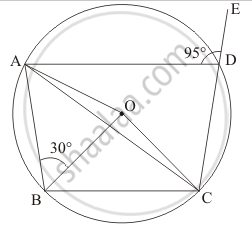Exercise 15.7 [Pages 109 - 112]

### RD Sharma solutions for Mathematics for Class 9 Chapter 15 Circles Exercise 15.7 [Pages 109 - 112]

Exercise 15.7 | Q 1 | Page 109

If the length of a chord of a circle is 16 cm and is at a distance of 15 cm from the centre of the circle, then the radius of the circle is

•  15 cm

• 16 cm

•  17 cm

•  34 cm

Exercise 15.7 | Q 2 | Page 109

The radius of a circle is 6 cm. The perpendicular distance from the centre of the circle to the chord which is 8 cm in length, is

• $\sqrt{5}$  cm

• $2\sqrt{5}$ cm

• $2\sqrt{7}$ cm

• $\sqrt{7}$  cm

Exercise 15.7 | Q 3 | Page 110

If O is the centre of a circle of radius r and AB is a chord of the circle at a distance r/2 from O, then ∠BAO =

•  60°

• 45°

•  30°

•  15°

Exercise 15.7 | Q 4 | Page 110

ABCD is a cyclic quadrilateral such that ∠ADB = 30° and ∠DCA = 80°, then ∠DAB =

•  70°

•  100°

•  125°

• 150°

Exercise 15.7 | Q 5 | Page 110

A chord of length 14 cm is at  a distance of 6 cm from the centre of a circle. The length of another chord at a distance of 2 cm from the centre of the circle is

•  12 cm

•  12 cm

•  12 cm

• 18 cm

Exercise 15.7 | Q 6 | Page 110

One chord of a circle is known to be 10 cm. The radius of this circle must be

•  5 cm

•  greater than 5 cm

• greater than or equal to 5 cm

• less than 5 cm

Exercise 15.7 | Q 7 | Page 110

ABC is a triangle with B as right angle, AC = 5 cm and AB = 4 cm. A circle is drawn with Aas centre and AC as radius. The length of the chord of this circle passing through C and B is

• 3 cm

• 4 cm

• 5 cm

• 6 cm

Exercise 15.7 | Q 8 | Page 110

If ABBC and CD are equal chords of a circle with O as centre and AD diameter, than ∠AOB =

•  60°

• 90°

• 120°

•  none of these

Exercise 15.7 | Q 9 | Page 110

Let C be the mid-point of an arc AB of a circle such that m $\stackrel\frown{AB}$  = 183°. If the region bounded by the arc ACB and the line segment AB is denoted by S, then the centre O of the circle lies

• in the interior of S

•  in the exertior of S

• on the segment AB

• on AB and bisects AB

Exercise 15.7 | Q 10 | Page 110

In a circle, the major arc is 3 times the minor arc. The corresponding central angles and the degree measures of two arcs are

•  90° and 270°

• 90° and 90°

•  270° and 90°

• 60° and 210°

Exercise 15.7 | Q 11 | Page 110

If A and B are two points on a circle such that m $\stackrel\frown{AB}$ = 260°. A possible value for the angle subtended by arc BA at a point on the circle is

• 100°

• 75°

•  50°

• 25°

Exercise 15.7 | Q 12 | Page 110

An equilateral triangle ABC is inscribed in a circle with centre O. The measures of ∠BOCis

• 30°

• 60°

•  90°

•  120°

Exercise 15.7 | Q 13 | Page 110

If two diameters of a circle intersect each other at right angles, then quadrilateral formed by joining their end points is a

• rhombus

•  rectangle

• parallelogram

•  square

Exercise 15.7 | Q 14 | Page 110

If ABC is an arc of a circle and ∠ABC = 135°, then the ratio of arc $\stackrel\frown{ABC}$ to the circumference is

•  1 : 4

• 3 : 4

•  3 : 8

• 1 : 2

Exercise 15.7 | Q 15 | Page 110

The chord of a circle is equal to its radius. The angle subtended by this chord at the minor arc of the circle is

•  60°

•  75°

•  120°

• 150°

Exercise 15.7 | Q 16 | Page 111

PQRS is a cyclic quadrilateral such that PR is a diameter of the circle. If ∠QPR = 67° and ∠SPR = 72°, then ∠QRS =

• 41°

•  23°

• 67°

• 18°

Exercise 15.7 | Q 17 | Page 111

If A , BC are three points on a circle with centre such that ∠AOB = 90° and ∠BOC = 120°, then ∠ABC =

•  60°

•  75°

• 90°

• 135°

Exercise 15.7 | Q 18 | Page 111

The greatest chord of a circle is called its

•  secant

• diameter

•  none of these

Exercise 15.7 | Q 19 | Page 111

Angle formed in minor segment of a circle is

• acute

•  obtuse

• right angle

• none of these

Exercise 15.7 | Q 20 | Page 111

Number of circles that can be drawn through three non-collinear points is

• 1

• 0

• 2

• 3

Exercise 15.7 | Q 21 | Page 111

In the given figure, if chords AB and CD of the circle intersect each other at right angles, then x + y =

•  45°

• 60°

• 75°

• 90°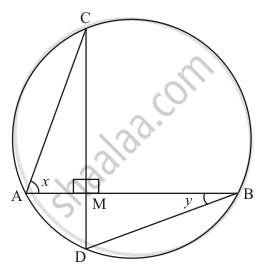Exercise 15.7 | Q 22 | Page 111

In the given figure, if ∠ABC = 45°, then ∠AOC =

• 45°

• 60°

• 75°

• 90°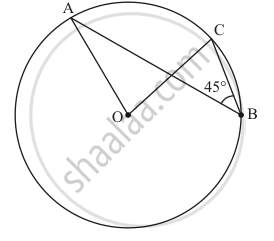Exercise 15.7 | Q 23 | Page 111

In the given figure, chords AD and BC intersect each other at right angles at a point P. If ∠DAB = 35°, then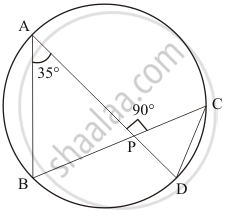•

35°

•  45°

• 55°

•  65°

Exercise 15.7 | Q 24 | Page 111

In the given figure, O is the centre of the circle and ∠BDC = 42°. The measure of ∠ACB is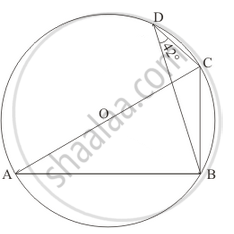•  42°

• 48°

•  58°

•  52°

Exercise 15.7 | Q 25 | Page 112

In a circle with centre O, AB and CD are two diameters perpendicular to each other. The length of chord AC is

• 2AB

• $\sqrt{2}$

• $\frac{1}{2}AB$

• $\frac{1}{\sqrt{2}}AB$

Exercise 15.7 | Q 26 | Page 112

Two equal circles of radius r intersect such that each passes through the centre of the other. The length of the common chord of the circle is

• $\sqrt{r}$

• $\sqrt{2}r AB$

• $\sqrt{3}r$

• $\frac{\sqrt{3}}{2}$

Exercise 15.7 | Q 27 | Page 112

If AB is a chord of a circle, P and Q are the two points on the circle different from A and B, then

• APB = ∠AQB

•  ∠APB + ∠AQB = 180° or ∠APB = ∠AQB

•  ∠APB + ∠AQB = 90°

•  ∠APB + ∠AQB = 180°

Exercise 15.7 | Q 28 | Page 112

AB and CD are two parallel chords of a circle with centre O such that AB = 6 cm and CD= 12 cm. The chords are on the same side of the centre and the distance between them is 3 cm. The radius of the circle is

• 6 cm

• $5\sqrt{2} cm$

•  7 cm

• $3\sqrt{5} cm$

Exercise 15.7 | Q 29 | Page 112

In a circle of radius 17 cm, two parallel chords are drawn on opposite side of a diameter. The distance between the chords is 23 cm. If the length of one chord is 16 cm, then the length of the other is

• 34 cm

• 15 cm

• 23 cm

• 30 cm

Exercise 15.7 | Q 30 | Page 112

In the given figure, O is the centre of the circle such that ∠AOC = 130°, then ∠ABC =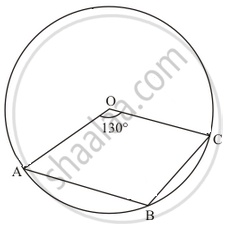• 130°

•  115°

• 65°

• 165°

## Chapter 15: Circles

Exercise 15.1Exercise 15.2Exercise 15.3Exercise 15.4Exercise 15.5Exercise 15.6Exercise 15.7## RD Sharma solutions for Mathematics for Class 9 chapter 15 - Circles

RD Sharma solutions for Mathematics for Class 9 chapter 15 (Circles) include all questions with solution and detail explanation. This will clear students doubts about any question and improve application skills while preparing for board exams. The detailed, step-by-step solutions will help you understand the concepts better and clear your confusions, if any. Shaalaa.com has the CBSE Mathematics for Class 9 solutions in a manner that help students grasp basic concepts better and faster.

Further, we at Shaalaa.com provide such solutions so that students can prepare for written exams. RD Sharma textbook solutions can be a core help for self-study and acts as a perfect self-help guidance for students.

Concepts covered in Mathematics for Class 9 chapter 15 Circles are Angle Subtended by a Chord at a Point, Perpendicular from the Centre to a Chord, Equal Chords and Their Distances from the Centre, Angle Subtended by an Arc of a Circle, Concept of Circle - Centre, Radius, Diameter, Arc, Sector, Chord, Segment, Semicircle, Circumference, Interior and Exterior, Concentric Circles, Cyclic Quadrilateral, Circles Passing Through One, Two, Three Points.

Using RD Sharma Class 9 solutions Circles exercise by students are an easy way to prepare for the exams, as they involve solutions arranged chapter-wise also page wise. The questions involved in RD Sharma Solutions are important questions that can be asked in the final exam. Maximum students of CBSE Class 9 prefer RD Sharma Textbook Solutions to score more in exam.

Get the free view of chapter 15 Circles Class 9 extra questions for Mathematics for Class 9 and can use Shaalaa.com to keep it handy for your exam preparation